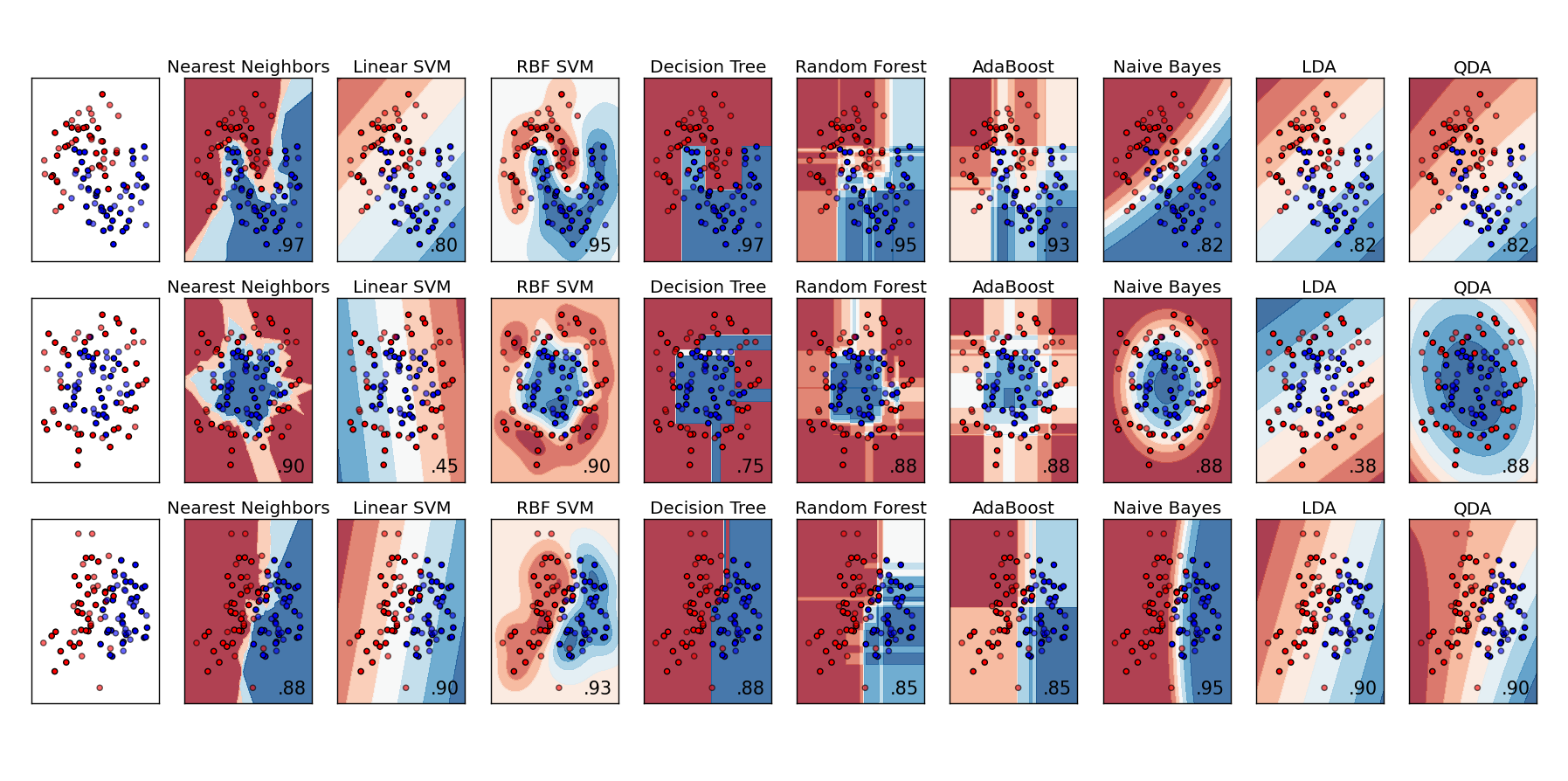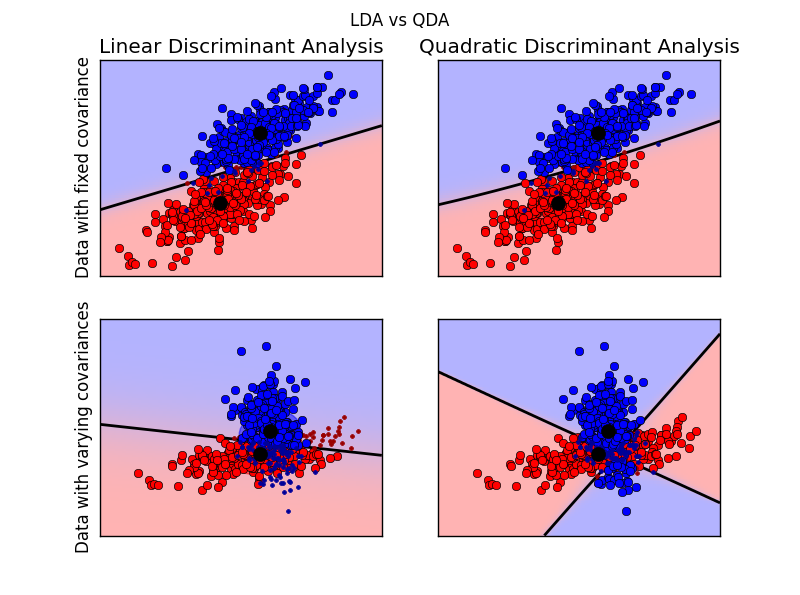• 【机器学习】Sklearn 常用分类器（全） ### KNN Classifier from sklearn.neighbors import KNeighborsClassifier clf = KNeighborsClassifier() clf.fit(train_x, train_y) __________________________________...
【机器学习】Sklearn 常用分类器（全）
### KNN Classifier
from sklearn.neighbors import KNeighborsClassifier

clf = KNeighborsClassifier()
clf.fit(train_x, train_y)
__________________________________________________________

### Logistic Regression Classifier
from sklearn.linear_model import LogisticRegression

clf = LogisticRegression(penalty='l2')
clf.fit(train_x, train_y)
__________________________________________________________

### Random Forest Classifier
from sklearn.ensemble import RandomForestClassifier

clf = RandomForestClassifier(n_estimators=8)
clf.fit(train_x, train_y)
__________________________________________________________

### Decision Tree Classifier
from sklearn import tree

clf = tree.DecisionTreeClassifier()
clf.fit(train_x, train_y)
__________________________________________________________

### GBDT(Gradient Boosting Decision Tree) Classifier

clf.fit(train_x, train_y)
__________________________________________________________

clf.fit(train_x, train_y)
__________________________________________________________

### GaussianNB
from sklearn.naive_bayes import GaussianNB

clf = GaussianNB()
clf.fit(train_x, train_y)
__________________________________________________________

### Linear Discriminant Analysis
from sklearn.discriminant_analysis import LinearDiscriminantAnalysis

clf = LinearDiscriminantAnalysis()
clf.fit(train_x, train_y)
__________________________________________________________

clf.fit(train_x, train_y)
__________________________________________________________

### SVM Classifier
from sklearn.svm import SVC

clf = SVC(kernel='rbf', probability=True)
clf.fit(train_x, train_y)
__________________________________________________________

### Multinomial Naive Bayes Classifier
from sklearn.naive_bayes import MultinomialNB

clf = MultinomialNB(alpha=0.01)
clf.fit(train_x, train_y)


展开全文• MATLAB中分类器有：K近邻分类器，随机森林分类器，朴素贝叶斯，集成学习方法，鉴别分析分类器，支持向量机。 现将其主要函数使用方法总结如下，更多细节需参考MATLAB 帮助文件。设： 　训练样本 ：train_data 　...

MATLAB中分类器有：K近邻分类器，随机森林分类器，朴素贝叶斯，集成学习方法，鉴别分析分类器，支持向量机。

现将其主要函数使用方法总结如下，更多细节需参考MATLAB 帮助文件。
设：  　　训练样本 ：train_data  　　训练样本标签：train_label  　　测试样本 ：test_data  　　测试样本标签：test_label
K近邻分类器 （KNN）
mdl = ClassificationKNN.fit(train_data,train_label,’NumNeighbors’,1);  predict_label = predict(mdl, test_data);  accuracy = length(find(predict_label == test_label))/length(test_label)*100
随机森林分类器（Random Forest）
B = TreeBagger(nTree,train_data,train_label);  predict_label = predict(B,test_data);
朴素贝叶斯 （Naive Bayes）
nb = NaiveBayes.fit(train_data, train_label);  predict_label = predict(nb, test_data);  accuracy = length(find(predict_label == test_label))/length(test_label)*100;
集成学习方法（Ensembles for Boosting, Bagging, or Random Subspace）
鉴别分析分类器（discriminant analysis classifier）
obj = ClassificationDiscriminant.fit(train_data, train_label);  predict_label = predict(obj, test_data);
支持向量机（Support Vector Machine, SVM）
SVMStruct = svmtrain(train_data, train_label);  predict_label = svmclassify(SVMStruct, test_data)
展开全文MATLAB 模式识别
• 常用分类器包括SVM、KNN、贝叶斯、线性回归、逻辑回归、决策树、随机森林、xgboost、GBDT、boosting、神经网络NN。 代码如下： from sklearn.metrics import precision_recall_fscore_support def ...


常用的分类器包括SVM、KNN、贝叶斯、线性回归、逻辑回归、决策树、随机森林、xgboost、GBDT、boosting、神经网络NN。

代码如下：

from

sklearn.metrics
import

precision_recall_fscore_support

def

timeDecor(func):

#一个用于统计函数运行时间的装饰器

def

innerDef(
*
args,
**
kwargs):

t1
=

time.time()

result
=

func(
*
args,
**
kwargs)

t2
=

time.time()

t
=

t2
-

t1

print

"{0}函数部分运行时间 ：{1}s"
.
format
(
str
(func.__name__),t)

return

result

return

innerDef

@timeDecor

def

svm_classify(X_train, y_train, X_test, y_test):

from

sklearn
import

svm

param_grid
=

{

# 'C': [1e3, 5e3, 1e4, 5e4, 1e5],

'kernel'
: [
'rbf'
,
'linear'
,
'poly'
,
'sigmoid'
],

# 'gamma': [0.0001, 0.0005, 0.001, 0.005, 0.01, 0.1],

}

t0
=

time()

clf
=

svm.SVC()

clf.fit(X_train, y_train)

# print(clf.best_params_)

print
(
"svm done in %0.3fs"

%

(time()
-

t0))

pre_y_train
=

clf.predict(X_train)

pre_y_test
=

clf.predict(X_test)

print
(
"SVM Metrics : {0}"
.
format
(precision_recall_fscore_support(y_test, pre_y_test)))

@timeDecor

def

rf_classify(X_train, y_train, X_test, y_test):

from

sklearn.ensemble
import

RandomForestClassifier

t0
=

time()

clf
=

RandomForestClassifier(random_state
=
0
, n_estimators
=
500
)

clf.fit(X_train, y_train)

print
(
"rf done in %0.3fs"

%

(time()
-

t0))

pre_y_train
=

clf.predict(X_train)

pre_y_test
=

clf.predict(X_test)

print
(
"rf Metrics : {0}"
.
format
(precision_recall_fscore_support(y_test, pre_y_test)))

@timeDecor

def

knn_classify(X_train, y_train, X_test, y_test):

from

sklearn.neighbors
import

KNeighborsClassifier

t0
=

time()

clf
=

KNeighborsClassifier(n_neighbors
=
5
)

clf.fit(X_train, y_train)

print
(
"knn done in %0.3fs"

%

(time()
-

t0))

pre_y_train
=

clf.predict(X_train)

pre_y_test
=

clf.predict(X_test)

print
(
"knn Metrics : {0}"
.
format
(precision_recall_fscore_support(y_test, pre_y_test)))

@timeDecor

def

bagging_knn_classify(X_train, y_train, X_test, y_test):

from

sklearn.neighbors
import

KNeighborsClassifier

from

sklearn.ensemble
import

BaggingClassifier

t0
=

time()

clf
=

BaggingClassifier(KNeighborsClassifier(),

max_samples
=
0.5
, max_features
=
0.5
)

clf.fit(X_train, y_train)

print
(
"bagging_knn done in %0.3fs"

%

(time()
-

t0))

pre_y_test
=

clf.predict(X_test)

print
(
"bagging_knn Metrics : {0}"
.
format
(precision_recall_fscore_support(y_test, pre_y_test)))

@timeDecor

def

lr_classify(X_train, y_train, X_test, y_test):

from

sklearn.linear_model
import

LogisticRegression

t0
=

time()

clf
=

LogisticRegression(C
=
1e5
)

clf.fit(X_train, y_train)

print
(
"lr done in %0.3fs"

%

(time()
-

t0))

pre_y_train
=

clf.predict(X_train)

pre_y_test
=

clf.predict(X_test)

print
(
"lr Metrics : {0}"
.
format
(precision_recall_fscore_support(y_test, pre_y_test)))

@timeDecor

def

nb_classify(X_train, y_train, X_test, y_test):

from

sklearn.naive_bayes
import

GaussianNB

t0
=

time()

clf
=

GaussianNB()

clf.fit(X_train, y_train)

print
(
"nb done in %0.3fs"

%

(time()
-

t0))

pre_y_train
=

clf.predict(X_train)

pre_y_test
=

clf.predict(X_test)

print
(
"nb Metrics : {0}"
.
format
(precision_recall_fscore_support(y_test, pre_y_test)))

@timeDecor

def

da_classify(X_train, y_train, X_test, y_test):

from

sklearn.discriminant_analysis
import

t0
=

time()

clf
=

clf.fit(X_train, y_train)

print
(
"da done in %0.3fs"

%

(time()
-

t0))

pre_y_test
=

clf.predict(X_test)

print
(
"da Metrics : {0}"
.
format
(precision_recall_fscore_support(y_test, pre_y_test)))

@timeDecor

def

decisionTree_classify(X_train, y_train, X_test, y_test):

from

sklearn.tree
import

DecisionTreeClassifier

t0
=

time()

clf
=

DecisionTreeClassifier(max_depth
=
5
)

clf.fit(X_train, y_train)

print
(
"DT done in %0.3fs"

%

(time()
-

t0))

pre_y_test
=

clf.predict(X_test)

print
(
"DT Metrics : {0}"
.
format
(precision_recall_fscore_support(y_test, pre_y_test)))

@timeDecor

def

xgboost_classify(X_train, y_train, X_test, y_test):

import

xgboost

t0
=

time()

clf
=

xgboost.XGBClassifier()

clf.fit(X_train, y_train)

print
(
"xgboost done in %0.3fs"

%

(time()
-

t0))

pre_y_test
=

clf.predict(X_test)

print
(
"xgboost Metrics : {0}"
.
format
(precision_recall_fscore_support(y_test, pre_y_test)))

@timeDecor

def

GBDT_classify(X_train, y_train, X_test, y_test):

from

sklearn.ensemble
import

t0
=

time()

clf
=

=
200
)

clf.fit(X_train, y_train)

print
(
"GBDT done in %0.3fs"

%

(time()
-

t0))

pre_y_test
=

clf.predict(X_test)

print
(
"GBDT Metrics : {0}"
.
format
(precision_recall_fscore_support(y_test, pre_y_test)))

@timeDecor

def

voting_classify(X_train, y_train, X_test, y_test):

from

sklearn.ensemble
import

import

xgboost

from

sklearn.linear_model
import

LogisticRegression

from

sklearn.naive_bayes
import

GaussianNB

t0
=

time()

clf1
=

=
200
)

clf2
=

RandomForestClassifier(random_state
=
0
, n_estimators
=
500
)

# clf3 = LogisticRegression(random_state=1)

# clf4 = GaussianNB()

clf5
=

xgboost.XGBClassifier()

clf
=

VotingClassifier(estimators
=
[

# ('gbdt',clf1),

(
'rf'
,clf2),

# ('lr',clf3),

# ('nb',clf4),

# ('xgboost',clf5),

],

voting
=
'soft'

)

clf.fit(X_train, y_train)

print
(
"voting done in %0.3fs"

%

(time()
-

t0))

pre_y_test
=

clf.predict(X_test)

print
(
"voting Metrics : {0}"
.
format
(precision_recall_fscore_support(y_test, pre_y_test)))


展开全文python 机器学习 算法
• 机器学习发展到现在，许多被证实有效的分类算法被提出，例如我们经常会用到的K-近邻分类器、朴素贝叶斯分类器、支持向量机(SVM)、决策树算法等。大家平时在用的时候可能并不太清楚每种分类算法适合哪种类型的数据，...
如果把机器学习归为两大类，那么主要的工作可以分为：分类和聚类。而分类任务基本上占整个机器学习或者是数据挖掘领域的70%,可见我们遇到的很多问题，都可以用分类的算法进行解决。机器学习发展到现在，许多被证实有效的分类算法被提出，例如我们经常会用到的K-近邻分类器、朴素贝叶斯分类器、支持向量机(SVM)、决策树算法等。大家平时在用的时候可能并不太清楚每种分类算法适合哪种类型的数据，因为对于不同的数据集，上述算法的效果可能有很大的区别，所以了解每种分类器的特点对于解决实际问题有很大的帮助。下图是我从网上找的一张典型的分类器性能对比图，我个人觉得很醒目，有一定的参考意义。大家可能对其中的LDA和QDA不太熟，我从百度文库中截取了它的相关介绍：

线性判别分析(Linear Discriminant Analysis, LDA)，有时也称Fisher线性判别(Fisher Linear Discriminant ,FLD)， 这种算法是Ronald Fisher 于 1936年发明的，是模式识别的经典算法。在1996年由Belhumeur引入模式识别和人工智能领域的。性鉴别分析的基本思想是将高维的模式样本投影到最佳鉴别矢量空间，以达到抽取分类信息和压缩特征空间维数的效果，投影后保证模式样本在新的子空间有最大的类间距离和最小的类内距离，即模式在该空间中有最佳的可分离性。因此，它是一种有效的特征抽取方法。使用这种方法能够使投影后模式样本的类间散布矩阵最大，并且同时类内散布矩阵最小。就是说，它能够保证投影后模式样本在新的空间中有最小的类内距离和最大的类间距离，即模式在该空间中有最佳的可分离性。展开全文• 传统的机器学习的监督学习分类分类和回归，分类是争对离散的数据，而回归是争对连续的数据，在数据预处理好的基础上要对数据进行预测，通常采用CV交叉验证来进行模型评价和选择。这篇文章通过连续的数据结合sklearn...
• 模式识别的几个经典分类器，附matlab程序。模式识别 分类器 贝叶斯 感知器 模式识别的几个经典分类器，附matlab程序。
• ## 机器学习中常见分类器的应用场景

万次阅读 多人点赞 2017-06-02 15:22:54
正好14年的时候有人做过一个实验，比较在不同数据集上（121个），不同的分类器（179个）的实际效果。 论文题为：Do we Need Hundreds of Classifiers to Solve Real World Classification Problems? 实验时间...
•keras
• 支持向量机之所以成为目前最常用，效果最好的分类器之一，在于其优秀的泛化能力，这是是因为其本身的优化目标是结构化风险最小，而不是经验风险最小，因此，通过margin的概念，得到对数据分布的结构化描述，因此减低...
• 一个分类器的分类准确率在60%-80%，即：比随机预测略好，但准确率却不太高，我们可以称之为“弱分类器”，比如CART（classification and regression tree分类与回归树）。 反之，如果分类精度90%以上，则是强分类...机器学习
• ## 贝叶斯分类器详解

万次阅读 多人点赞 2019-07-07 08:22:20
本文介绍了贝叶斯的基本知识，然后给出了极大似然估计。最后给出了朴素贝叶斯分类器和半朴素贝叶斯分类器，并给出了西瓜书上相关的例题。机器学习 极大似然估计
• ## 理解高斯分类器

千次阅读 2019-06-20 08:37:13
在本文中，我们讨论了单变量和多变量正态分布，以及如何使用贝叶斯定理导出高斯分类器。像高斯这样的分类器简单而直观，并且可以解释。 正态/高斯分布 在现实生活中，我们总是会对某些现象产生不确定性...
• 这篇学习文章是在上一篇博客（http://blog.csdn.net/july_sun/article/details/53088673）的基础上，从机器学习的四要素（数据，算法和模型，计算机硬件，机器学习平台）角度出发用实例将各个分类器做一比较.机器学习
• 本文大致分成两大部分，第一部分尝试将本文涉及的分类器统一到神经元类模型中，第二部分阐述卷积神经网络（CNN）的发展简述和目前的相关工作。 本文涉及的分类器（分类方法）有： 线性回归逻辑回归（即神经元...深度学习 神经网络
• 在人脸识别中有几种常用分类器，一是最邻近分类器；二是线性分类器 （1）最邻近分类器  最近邻分类器是模式识别领域中最常用的分类方法之一，其直观简单，在通常的应用环境中非常有效。因此在人脸识别问题中...
• ## 分类器

万次阅读 多人点赞 2018-09-21 21:23:10
分类器的作用：常规任务是利用给定的类别、已知的训练数据来学习分类规则和分类器，然后对未知数据进行分类（或预测）。逻辑回归（logistics）、SVM等常用于解决二分类问题，对于多分类问题（multi-class ...
• 朴素贝叶斯分类器是分类算法集合中基于贝叶斯理论的一种算法。它不是单一存在的，而是一个算法家族，在这个算法家族中它们都有共同的规则。例如每个被分类的特征对与其他的特征对都是相互独立的。 朴素贝叶斯分类器...
• 目录常见特征提取方法基于EEG的脑机接口分类算法 人工智能的发展也给脑机接口技术带来了很广阔的空间，目前限制脑机接口技术的走出实验室的...除上述两种常用的特征提取方法之外的方法： 1、连接特征，这些特征测...脑机接口
• adaboost弱分类器与强分类器的训练过程（偏理论） 具体步骤如下： 1.初始化样本权重D1=1/(n+m) 2.对每个特征f（20*20中有78460个特征），计算所有样本（正样本n个，负样本m个）的特征值。 3.将每个特征对应的m+n个...adaboost
• ## 遥感图像分类

万次阅读 多人点赞 2019-01-28 21:36:02
遥感图像分类 一、背景简介 遥感图像分类就是利用计算机通过对遥感图像中各类地物的光谱信息和空间信息进行分析，选择特征，将图像中各个像元按照某种规则或算法划分不同的类别，然后获得遥感图像中与实际地物的...遥感图像
• K-means算法以欧式距离作为相似度测度，它是求对应某一初始聚类中心向量V最优分类，使得评价指标J最小。算法采用误差平方和准则函数作为聚类准则函数。 该算法是典型的基于聚类的聚类算法，采用距离作为相似性的评价...
• 本文主要介绍图像的线性分类器。首先介绍感知机，并由感知机引出评价函数与代价函数两个概念；比较了SVM 与 Softmax 两类分类器的区别。感知机 SVM Softmax 损失函数
• 本课程在技术栈层面涵盖了前端和后端的大部分常用技术，包括Spring Boot、Spring MVC、Mybatis、Mybatis-Plus、Shiro(身份认证与资源授权跟会话等等)、Spring AOP、防止XSS攻击、防止SQL注入攻击、过滤Filter、...java java面试题 java面试 java学习路线
• Matlab中常用分类器有随机森林分类器、支持向量机（SVM）、K近邻分类器、朴素贝叶斯、集成学习方法和鉴别分析分类器等。各分类器的相关Matlab函数使用方法如下：首先对以下介绍中所用到的一些变量做统一的说明： ...机器学习 Matlab
• 常用分类算法总结分类算法总结NBC算法LR算法SVM算法ID3算法C4.5 算法C5.0算法KNN 算法ANN 算法 分类算法总结 分类是在一群已经知道类别标号的样本中，训练一种分类器，让其能够对某种未知的样本进行分类。分类算法...
• Halcon中常用分类器有GMM（高斯混合模型）、Neural Nets（神经网络）、SVM（支持向量机）等。一般应付常见的分类问题，这些就足够了。 使用方法 一般使用过程： 创建分类器（create_class_…） 获取各个类别...halcon
• k-Nearest Neighbor分类器存在以下不足： 分类器必须记住所有训练数据并将其存储起来，以便于未来测试数据用于比较。这在存储空间上是低效的，数据集的大小很容易就以GB计。 对一个测试图像进行分类需要和所有...
• ## 理解贝叶斯分类器原理及关系

千次阅读 多人点赞 2019-01-01 19:42:01
贝叶斯分类器是什么？有什么用？怎么用？ 为了更好地理解这个问题，我们先看一个案例： 案例（来源于西瓜书）： 判断一个具有特征:{色泽=青绿，根蒂=蜷缩，敲声=浊响，纹理=清晰，脐部=凹陷，触感=硬...贝叶斯网络 机器学习
• 1 影响一个分类器错误率的因素 　(1)、训练集的记录数量。生成器要利用训练集进行学习，因而训练集越大，分类器也就越可靠。然而，训练集越大，生成器构造分类器的时间也就越长。错误率改善情况随训练集规模的增大而......